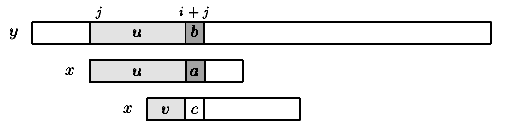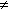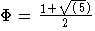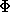Name
• String
• Substring
• Search
Edit
The design of the Knuth-Morris-Pratt algorithm follows a tight analysis of the Morris and Pratt algorithm. Let us look more closely at the Morris-Pratt algorithm. It is possible to improve the length of the shifts.

Consider an attempt at a left position j, that is when the the window is positioned on the text factor y[j .. j+m-1]. Assume that the first mismatch occurs between x[i] and y[i+j] with 0 < i < m. Then, x[0 .. i-1] = y[j .. i+j-1] =u and a = x[i]  y[i+j]=b.

When shifting, it is reasonable to expect that a prefix v of the pattern matches some suffix of the portion u of the text. Moreover, if we want to avoid another immediate mismatch, the character following the prefix v in the pattern must be different from a. The longest such prefix v is called the tagged border of u (it occurs at both ends of u followed by different characters in x).

This introduces the notation: let kmpNext[i] be the length of the longest border of x[0 .. i-1] followed by a character c different from x[i] and -1 if no such tagged border exits, for 0 < i  m. Then, after a shift, the comparisons can resume between characters x[kmpNext[i]] and y[i+j] without missing any occurrence of x in y, and avoiding a backtrack on the text (see figure 7.1). The value of kmpNext is set to -1.Figure 7.1: Shift in the Knuth-Morris-Pratt algorithm (v border of u and cb).

The table kmpNext can be computed in O(m) space and time before the searching phase, applying the same searching algorithm to the pattern itself, as if x=y.

The searching phase can be performed in O(m+n) time. The Knuth-Morris-Pratt algorithm performs at most 2n-1 text character comparisons during the searching phase. The delay (maximal number of comparisons for a single text character) is bounded by log(m) where  is the golden ratio ().

Main features:

• performs the comparisons from left to right;
• preprocessing phase in O(m) space and time complexity;
• searching phase in O(n+m) time complexity (independent from the alphabet size);
• delay bounded by log(m) whereis the golden ratio ().

C